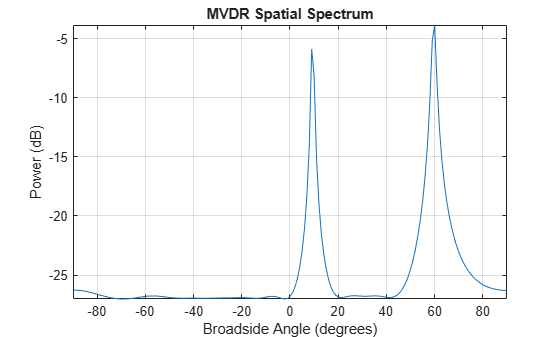# step

System object: phased.MVDREstimator
Package: phased

Perform spatial spectrum estimation

## Syntax

```Y = step(H,X) [Y,ANG] = step(H,X) ```

## Description

Note

Starting in R2016b, instead of using the `step` method to perform the operation defined by the System object™, you can call the object with arguments, as if it were a function. For example, ```y = step(obj,x)``` and `y = obj(x)` perform equivalent operations.

`Y = step(H,X)` estimates the spatial spectrum from `X` using the estimator `H`. `X` is a matrix whose columns correspond to channels. `Y` is a column vector representing the magnitude of the estimated spatial spectrum.

The size of the first dimension of the input matrix can vary to simulate a changing signal length. A size change can occur, for example, in the case of a pulse waveform with variable pulse repetition frequency.

`[Y,ANG] = step(H,X)` returns additional output `ANG` as the signal’s direction of arrival (DOA) when the `DOAOutputPort` property is true. `ANG` is a row vector of the estimated broadside angles (in degrees).

Note

The object performs an initialization the first time the object is executed. This initialization locks nontunable properties and input specifications, such as dimensions, complexity, and data type of the input data. If you change a nontunable property or an input specification, the System object issues an error. To change nontunable properties or inputs, you must first call the `release` method to unlock the object.

## Examples

expand all

First, estimate the DOAs of two signals received by a standard 10-element ULA with element spacing of 1 meter. The antenna operating frequency is 150 MHz. The actual direction of the first signal is 10° in azimuth and 20° in elevation. The direction of the second signal is 60° in azimuth and −5° in elevation. Then, plot the MVDR spatial spectrum.

Note: This example runs only in R2016b or later. If you are using an earlier release, replace each call to the function with the equivalent `step` syntax. For example, replace `myObject(x)` with `step(myObject,x)`.

Create the signals with added noise. Then, create the ULA System object™.

```fs = 8000; t = (0:1/fs:1).'; x1 = cos(2*pi*t*300); x2 = cos(2*pi*t*400); array = phased.ULA('NumElements',10,'ElementSpacing',1); array.Element.FrequencyRange = [100e6 300e6]; fc = 150.0e6; x = collectPlaneWave(array,[x1 x2],[10 20;60 -5]',fc); noise = 0.1*(randn(size(x)) + 1i*randn(size(x)));```

Construct MVDR estimator System object.

```estimator = phased.MVDREstimator('SensorArray',array,... 'OperatingFrequency',fc,'DOAOutputPort',true,'NumSignals',2);```

Estimate the DOAs.

```[y,doas] = estimator(x + noise); doas = broadside2az(sort(doas),[20 -5])```
```doas = 1×2 9.5829 60.3813 ```

Plot the spectrum.

`plotSpectrum(estimator)`